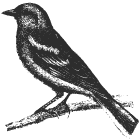# Pictures To Text

Mar 2019

Converted a picture into text just for fun. To complete this I used jupyter notebook, found below. The code is avaliable on github.

``````.gMBy
WgM_
M@DMg_
\$BMGDMg
`MgZMMg_
_<V _MMK_
_1P"`    "gg
``````

The notebook contains 3 parts:

1. Image - Input image to be converted. It’s chopped up into little bits.
2. Font - Input font. The characters are extrated as images.
3. Calc - the images and font characters are compared to find the best match
``````from PIL import Image, ImageDraw, ImageFont
import numpy as np
import matplotlib.pyplot as plt
from skimage.util.shape import view_as_blocks
``````

## Image

First thing is import the image. As an example I use the finch. Most of the following code is to convert the image from a PIL array to numpy’s 2D narray and use matlibplot plot function.

``````def show_image(path):
image = Image.open(path)
image = image.convert('L')
d = list(image.getdata())
image_2D = np.reshape(d, (image.height, image.width))
plt.imshow(image_2D, cmap='gray')

``````

Output:The next step is taking the imported image and converting it into character sized images. The heart of the following methods are the view_as_blocks. The function fails if there is an overlap with the edge of the imported image. The function trim_image is there to remove the excess before it sent in to view_as_blocks. The result can be seen below.

``````def trim_image(matrix, size_x, size_y):
height, width = matrix.shape
trim_height = height % size_y
trim_width = width % size_x

if trim_height > 0:
matrix = matrix[:-trim_height,...]

if trim_width > 0:
matrix = matrix[...,:-trim_width]

return matrix

def get_image_slices(image_path, char_width, char_height):
image = Image.open(image_path)
image = image.convert('L') #greyscale

data = list(image.getdata())
image_2D = np.reshape(data, (image.height, image.width))

image_2D = trim_image(image_2D, char_width, char_height)
image_slices = view_as_blocks(image_2D, block_shape=(char_height,char_width))

return image_slices
``````
``````char_width = 10
char_height = 18

images = image_slices.reshape(image_slices.shape*image_slices.shape,
image_slices.shape, image_slices.shape)
nb_across = image_slices.shape
nb_down = image_slices.shape
plt.figure()
for i, img in enumerate(images):
plt.subplot(nb_across, nb_down, i+1)
plt.imshow(img, cmap='gray', vmin=0, vmax=255)
``````

Output:## Fonts

Now we have the images, we can match characters to them. First we need to get images of the font to compare. We will be using the ascii character set, this is found in the string.printable function.

``````def font_images():
fnt = ImageFont.truetype('fonts/SFMono-Regular.otf', 15)
import string
letters = string.printable
results = []

for letter in letters:
img = Image.new('RGB', (10, 18), color=(255,255,255))
d = ImageDraw.Draw(img)
d.text((0,0),letter, font=fnt, fill=(0,0,0))
img = img.convert('L') #greyscale

d = list(img.getdata())
image_2D = np.reshape(d, (img.height, img.width))

results.append(image_2D)

return (letters, np.stack(results, axis=0))

``````
``````letters_idx, letters = font_images()
``````
``````plt.figure(figsize=(60,60))
for i, img in enumerate(letters):
plt.subplot(20, 20, i+1)
plt.imshow(img, cmap='gray', vmin=0, vmax=255)
``````

Output:## Calc

Now we have sections of the input image and character images, we just need to pick the best match. In this case I used:where A and B are matricis of the same size. We want the lowest score as that will be the smallest difference. I guess I could have inverted it so we were looking for the max or I could have called it the loss function.

``````def solve_section(section, letters):
min_score = 1000000
min_letter = None
id = 0

for letter in letters:
score = np.sum(np.absolute(letter - section))
if score < min_score:
min_score = score
min_letter = letter
min_id = id
id += 1

return (min_id, min_letter)
``````
``````def solve(sections, letters):
results = []
ids = []
for s in sections:
id, min_letter = solve_section(s, letters)
results.append(min_letter)
ids.append(id)

return (ids, np.stack(results, axis=0))
``````
``````ids, result = solve(f, letters)
``````
``````fig = plt.figure(figsize=(20, 20))

for i in range(len(result)):
sub.imshow(result[i], cmap='gray', vmin=0, vmax=255)
``````

Output:Now we can finish it by collecting the characters and printing

``````result_string = ""
i = 1
for id in ids:
result_string += letters_idx[id]
if i % image_slices.shape == 0:
result_string += '\n'
i += 1
``````
``````print(result_string)
``````

Output:

``````.gMBy
WgM_
M@DMg_
\$BMGDMg
`MgZMMg_
_<V _MMK_
_1P"`    "gg
``````